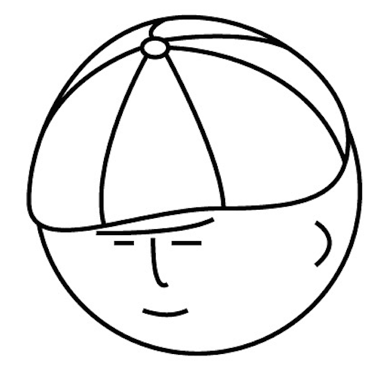マツシタのお勉強メモ

マツシタのお勉強

welcome to my engineering blog
Hacker RankFind the next node (in-oder traversal) of a given node in a binary search tree

Problem Write an algorithm to find the next node (i.e., in-oder successor) of a given node in a binary search tree. You may assume that each node has a link to its parent. Solution This problem can be solved by thinking the two patterns. F…

Find the First Common Ancestor of the two nodes in a binary tree

Problem Design an algorithm and write code to find the first common ancestor of two nodes in a binary tree. Avoid storing additional nodes in a data structure. Node: This is not necessarily a binary search tree. Solution I’ll solve this pr…